# Selina Solutions Concise Maths Class 10 Chapter 17 Circles Exercise 17(C)

The problems of this exercise strengthen the main concepts of the chapter. The Selina Solutions for Class 10 Maths is a one-stop solution to all the doubts and clarifications pertaining to solving problems of the book. The solutions PDF of the Concise Selina Solutions for Class 10 Maths Chapter 17 Circles Exercise 17(C) is available in the link given below.

## Selina Solutions Concise Maths Class 10 Chapter 17 Circles Exercise 17(C) Download PDF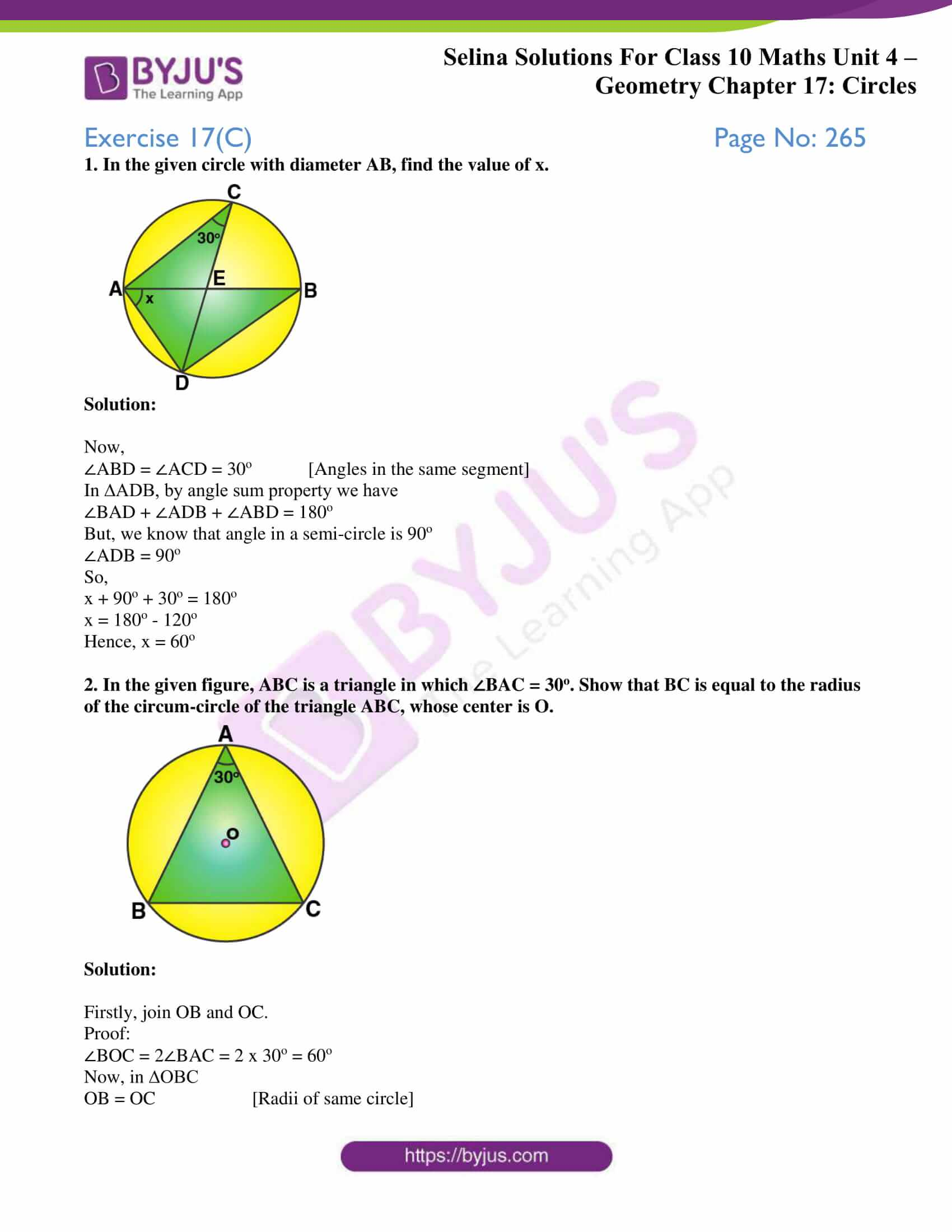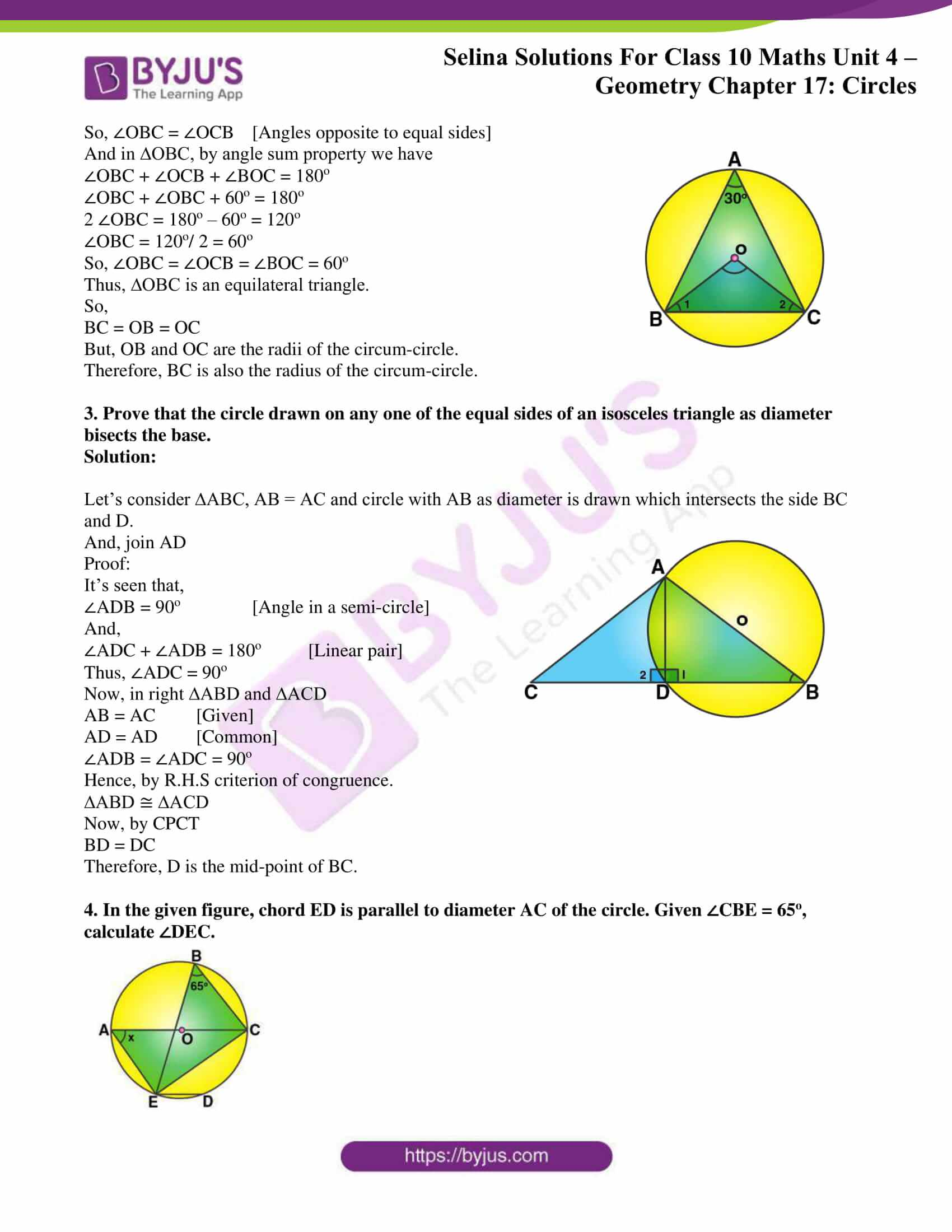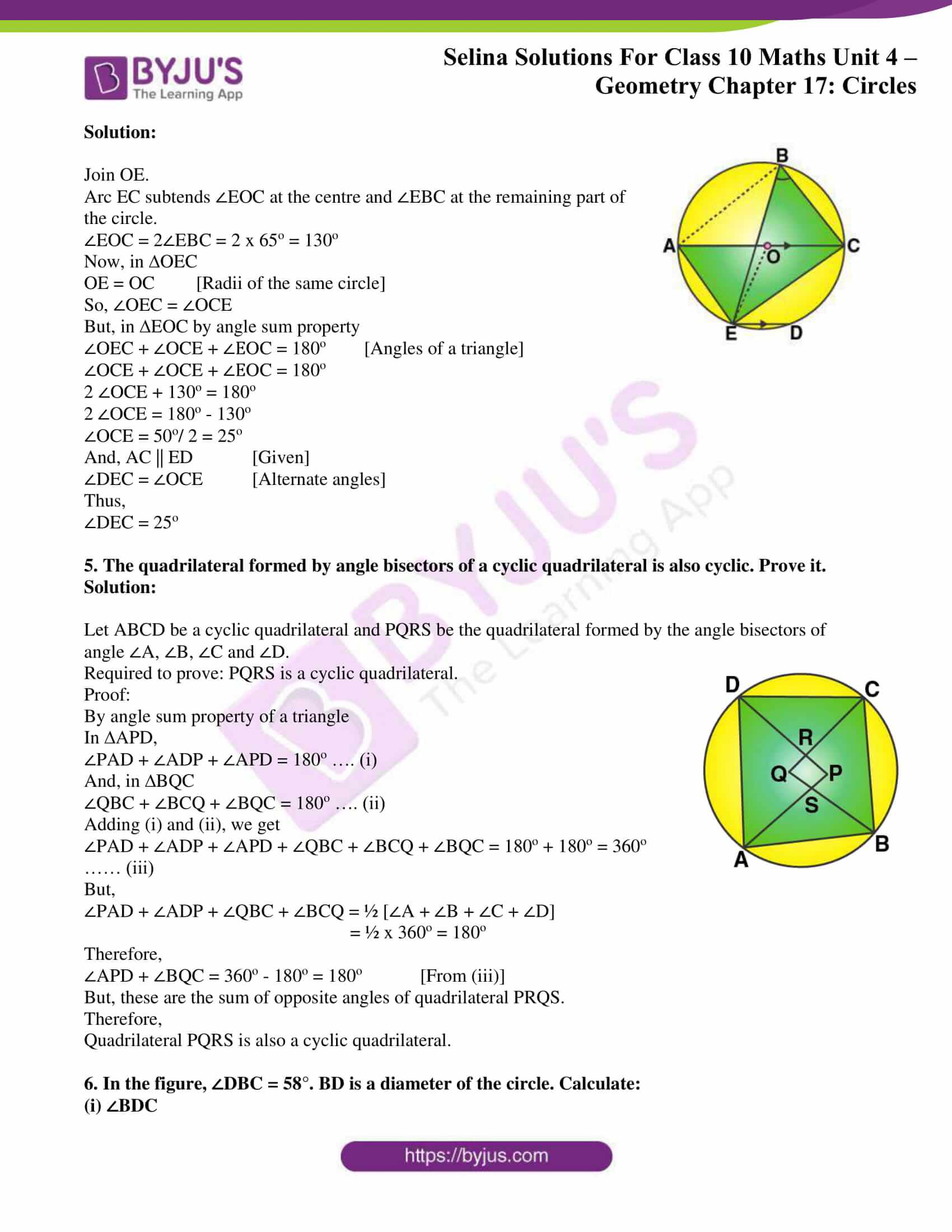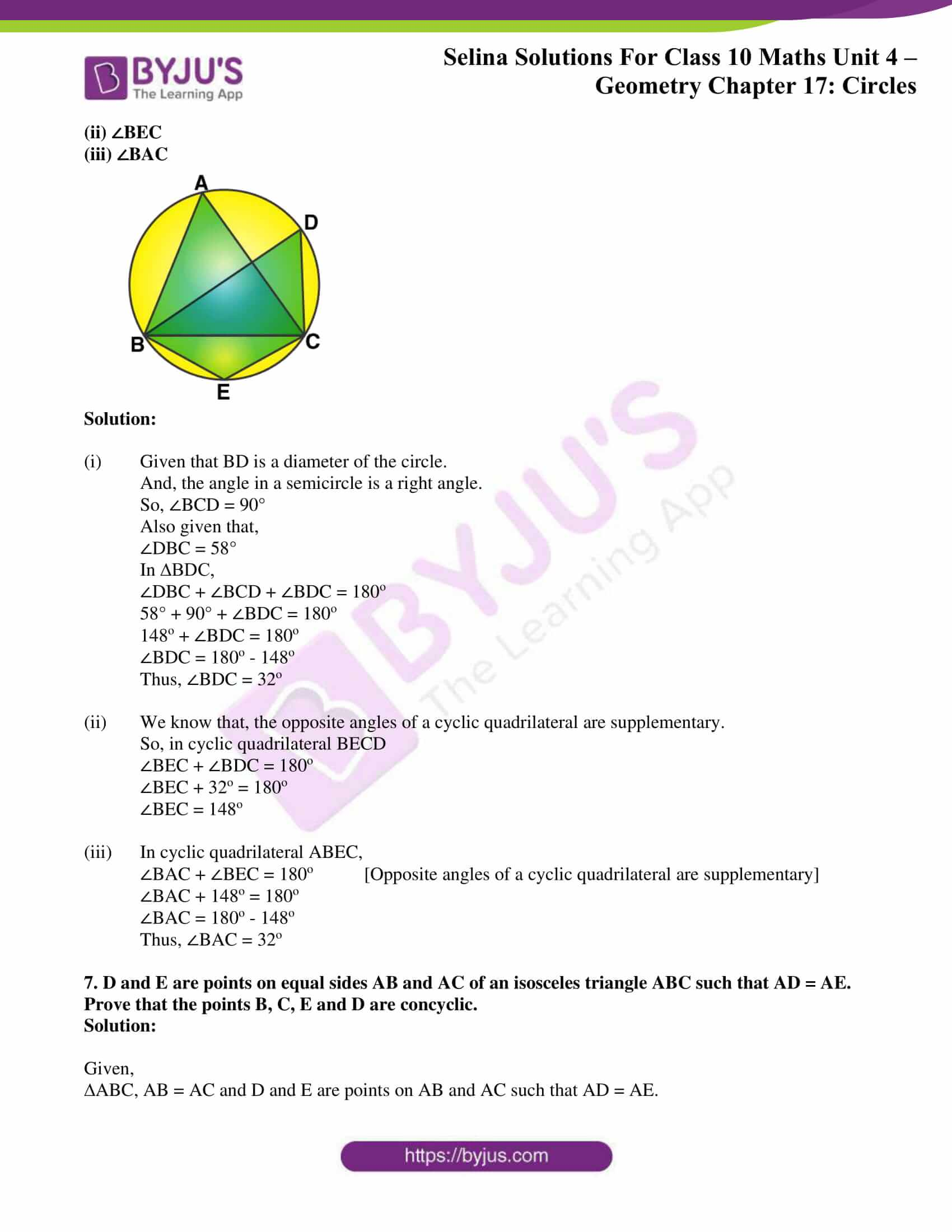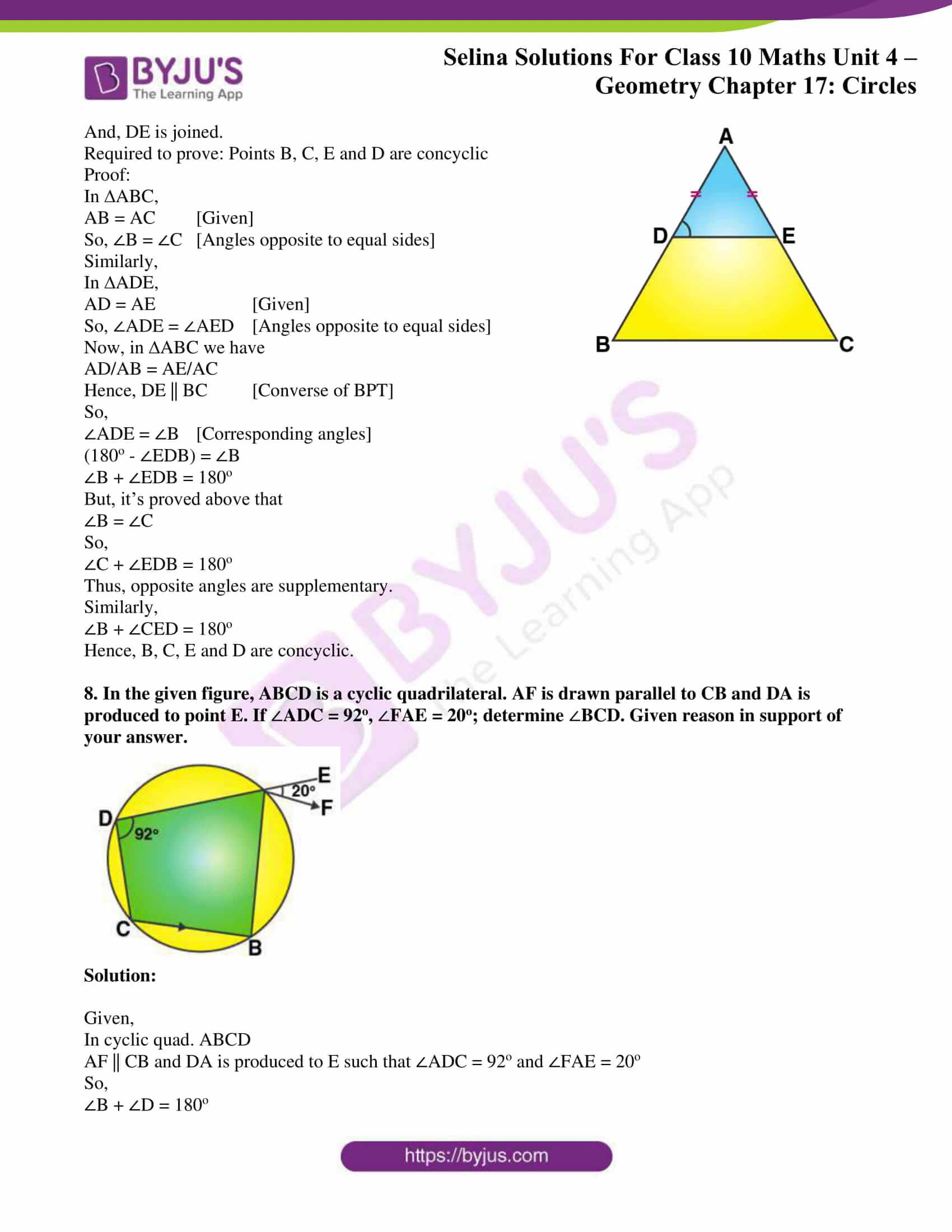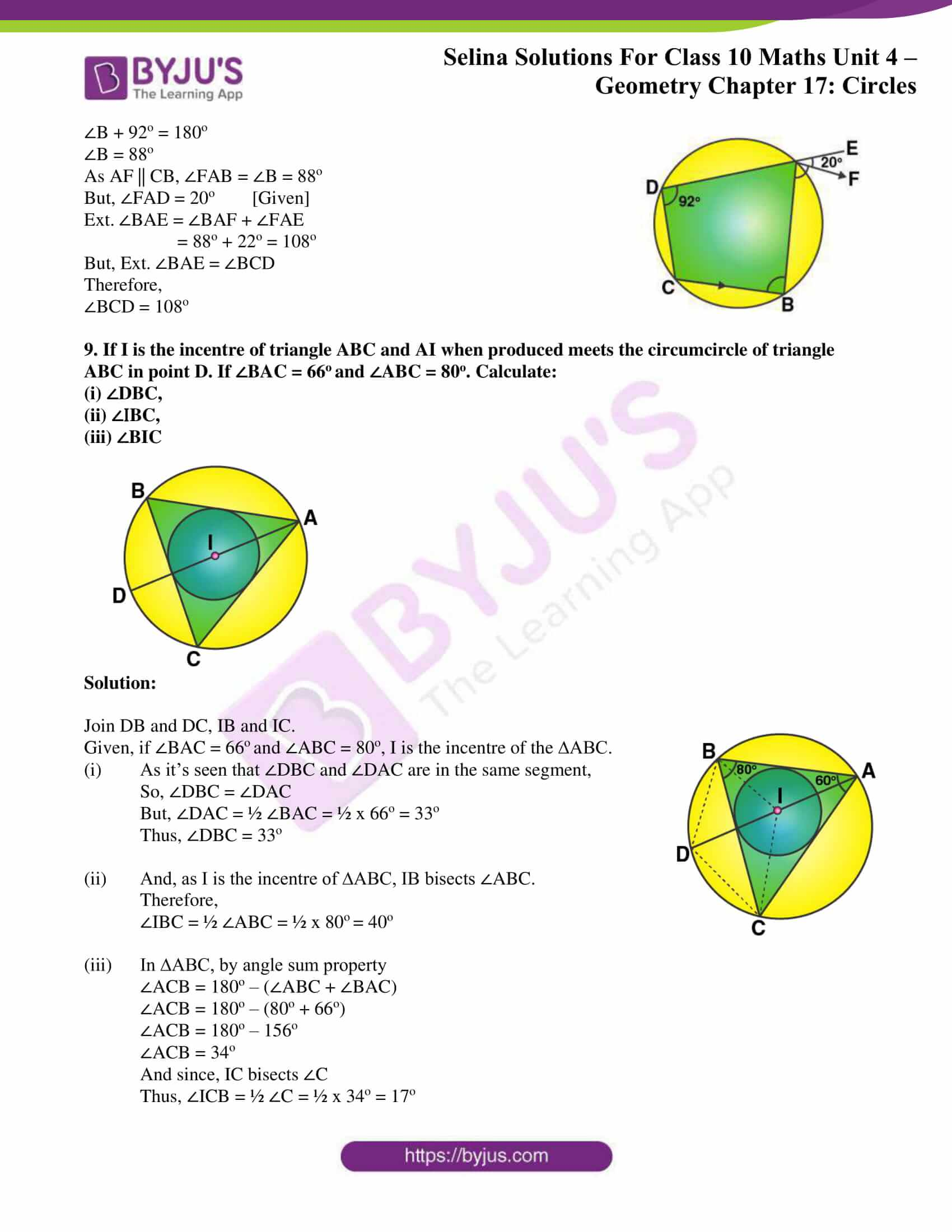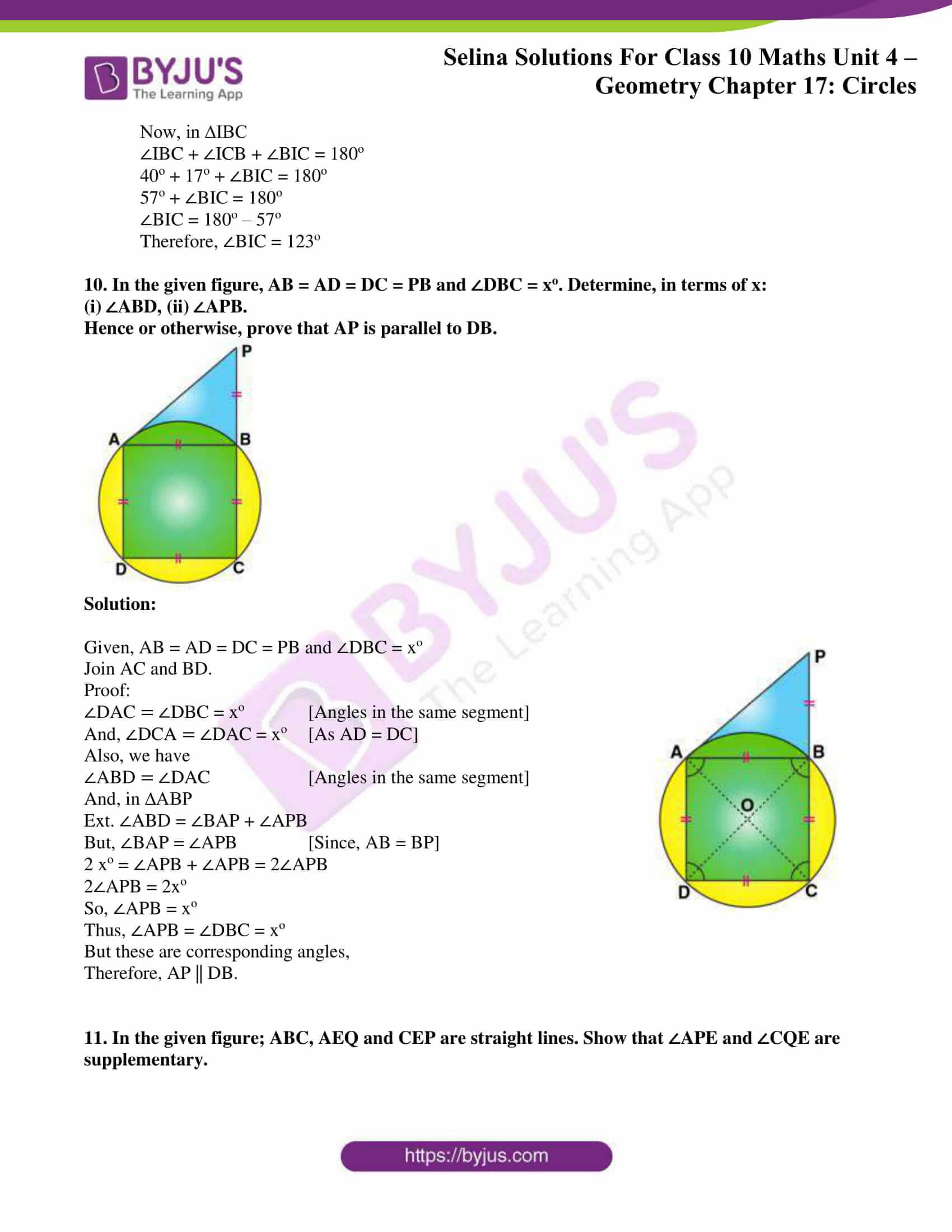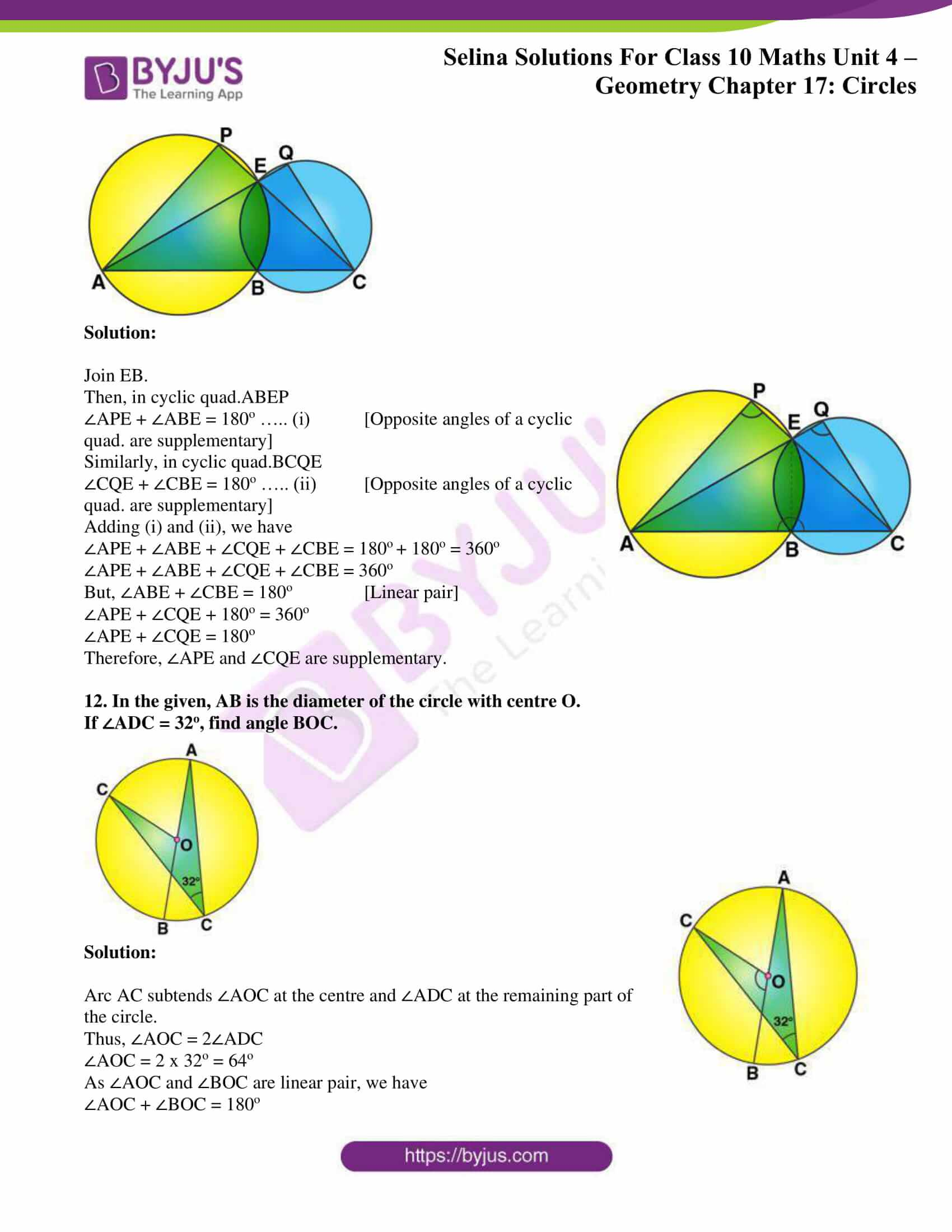### Access Selina Solutions Concise Maths Class 10 Chapter 17 Circles Exercise 17(C)

1. In the given circle with diameter AB, find the value of x.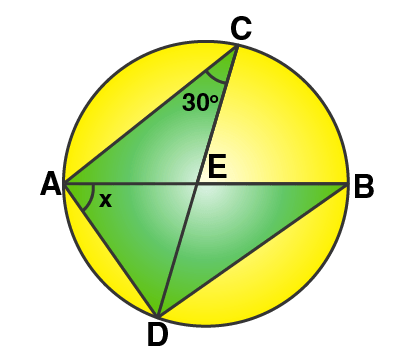Solution:

Now,

∠ABD = ∠ACD = 30o [Angles in the same segment]

In ∆ADB, by angle sum property we have

But, we know that angle in a semi-circle is 90o

So,

x + 90o + 30o = 180o

x = 180o – 120o

Hence, x = 60o

2. In the given figure, ABC is a triangle in which ∠BAC = 30o. Show that BC is equal to the radius of the circum-circle of the triangle ABC, whose center is O.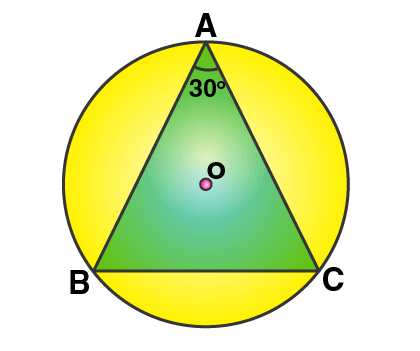Solution:

Firstly, join OB and OC.

Proof:

∠BOC = 2∠BAC = 2 x 30o = 60o

Now, in ∆OBC

OB = OC [Radii of same circle]

So, ∠OBC = ∠OCB [Angles opposite to equal sides]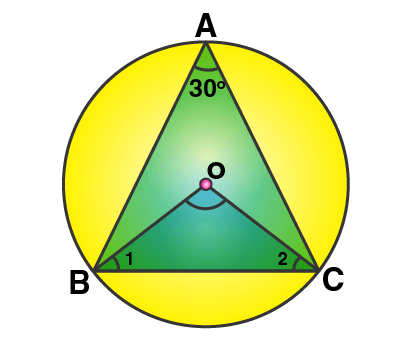And in ∆OBC, by angle sum property we have

∠OBC + ∠OCB + ∠BOC = 180o

∠OBC + ∠OBC + 60o = 180o

2 ∠OBC = 180o – 60o = 120o

∠OBC = 120o/ 2 = 60o

So, ∠OBC = ∠OCB = ∠BOC = 60o

Thus, ∆OBC is an equilateral triangle.

So,

BC = OB = OC

But, OB and OC are the radii of the circum-circle.

Therefore, BC is also the radius of the circum-circle.

3. Prove that the circle drawn on any one of the equal sides of an isosceles triangle as diameter bisects the base.

Solution: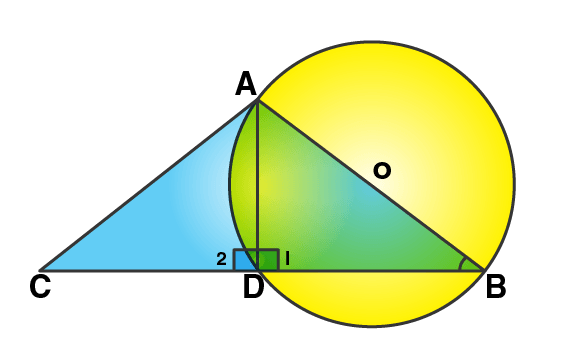Let’s consider ∆ABC, AB = AC and circle with AB as diameter is drawn which intersects the side BC and D.

Proof:

It’s seen that,

∠ADB = 90o [Angle in a semi-circle]

And,

Now, in right ∆ABD and ∆ACD

AB = AC [Given]

Hence, by R.H.S criterion of congruence.

∆ABD ≅ ∆ACD

Now, by CPCT

BD = DC

Therefore, D is the mid-point of BC.

4. In the given figure, chord ED is parallel to diameter AC of the circle. Given ∠CBE = 65o, calculate ∠DEC.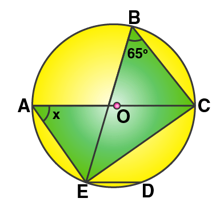Solution: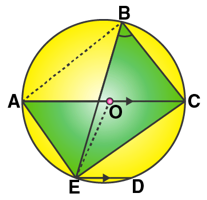Join OE.

Arc EC subtends ∠EOC at the centre and ∠EBC at the remaining part of the circle.

∠EOC = 2∠EBC = 2 x 65o = 130o

Now, in ∆OEC

OE = OC [Radii of the same circle]

So, ∠OEC = ∠OCE

But, in ∆EOC by angle sum property

∠OEC + ∠OCE + ∠EOC = 180o [Angles of a triangle]

∠OCE + ∠OCE + ∠EOC = 180o

2 ∠OCE + 130o = 180o

2 ∠OCE = 180o – 130o

∠OCE = 50o/ 2 = 25o

And, AC || ED [Given]

∠DEC = ∠OCE [Alternate angles]

Thus,

∠DEC = 25o

5. The quadrilateral formed by angle bisectors of a cyclic quadrilateral is also cyclic. Prove it.

Solution: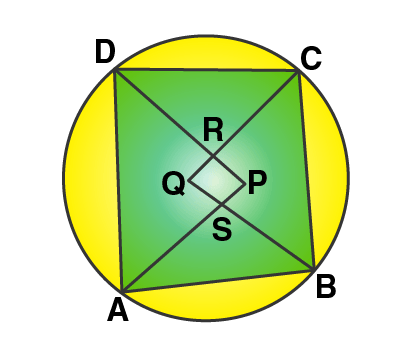Let ABCD be a cyclic quadrilateral and PQRS be the quadrilateral formed by the angle bisectors of angle ∠A, ∠B, ∠C and ∠D.

Required to prove: PQRS is a cyclic quadrilateral.

Proof:

By angle sum property of a triangle

In ∆APD,

And, in ∆BQC

∠QBC + ∠BCQ + ∠BQC = 180o …. (ii)

Adding (i) and (ii), we get

∠PAD + ∠ADP + ∠APD + ∠QBC + ∠BCQ + ∠BQC = 180o + 180o = 360o …… (iii)

But,

∠PAD + ∠ADP + ∠QBC + ∠BCQ = ½ [∠A + ∠B + ∠C + ∠D]

= ½ x 360o = 180o

Therefore,

∠APD + ∠BQC = 360o – 180o = 180o [From (iii)]

But, these are the sum of opposite angles of quadrilateral PRQS.

Therefore,

6. In the figure, ∠DBC = 58°. BD is a diameter of the circle. Calculate:

(i) ∠BDC

(ii) ∠BEC

(iii) ∠BAC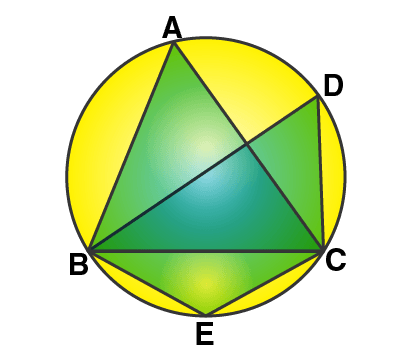Solution:

(i) Given that BD is a diameter of the circle.

And, the angle in a semicircle is a right angle.

So, ∠BCD = 90°

Also given that,

∠DBC = 58°

In ∆BDC,

∠DBC + ∠BCD + ∠BDC = 180o

58° + 90° + ∠BDC = 180o

148o + ∠BDC = 180o

∠BDC = 180o – 148o

Thus, ∠BDC = 32o

(ii) We know that, the opposite angles of a cyclic quadrilateral are supplementary.

∠BEC + ∠BDC = 180o

∠BEC + 32o = 180o

∠BEC = 148o

∠BAC + ∠BEC = 180o [Opposite angles of a cyclic quadrilateral are supplementary]

∠BAC + 148o = 180o

∠BAC = 180o – 148o

Thus, ∠BAC = 32o

7. D and E are points on equal sides AB and AC of an isosceles triangle ABC such that AD = AE. Prove that the points B, C, E and D are concyclic.

Solution:

Given,

∆ABC, AB = AC and D and E are points on AB and AC such that AD = AE.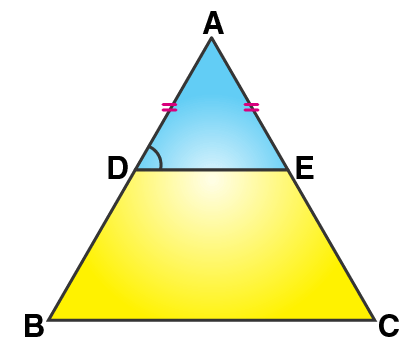And, DE is joined.

Required to prove: Points B, C, E and D are concyclic

Proof:

In ∆ABC,

AB = AC [Given]

So, ∠B = ∠C [Angles opposite to equal sides]

Similarly,

So, ∠ADE = ∠AED [Angles opposite to equal sides]

Now, in ∆ABC we have

Hence, DE || BC [Converse of BPT]

So,

(180o – ∠EDB) = ∠B

∠B + ∠EDB = 180o

But, it’s proved above that

∠B = ∠C

So,

∠C + ∠EDB = 180o

Thus, opposite angles are supplementary.

Similarly,

∠B + ∠CED = 180o

Hence, B, C, E and D are concyclic.

8. In the given figure, ABCD is a cyclic quadrilateral. AF is drawn parallel to CB and DA is produced to point E. If ADC = 92o, FAE = 20o; determine BCD. Given reason in support of your answer.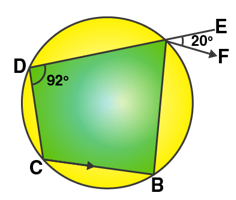Solution:

Given,

AF || CB and DA is produced to E such that ∠ADC = 92o and ∠FAE = 20o

So,

∠B + ∠D = 180o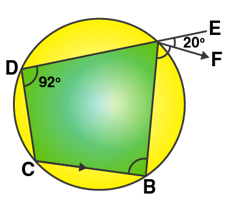∠B + 92o = 180o

∠B = 88o

As AF || CB, ∠FAB = ∠B = 88o

Ext. ∠BAE = ∠BAF + ∠FAE

= 88o + 22o = 108o

But, Ext. ∠BAE = ∠BCD

Therefore,

∠BCD = 108o

9. If I is the incentre of triangle ABC and AI when produced meets the circumcircle of triangle ABC in point D. If ∠BAC = 66o and ∠ABC = 80o. Calculate:

(i) ∠DBC,

(ii) ∠IBC,

(iii) ∠BIC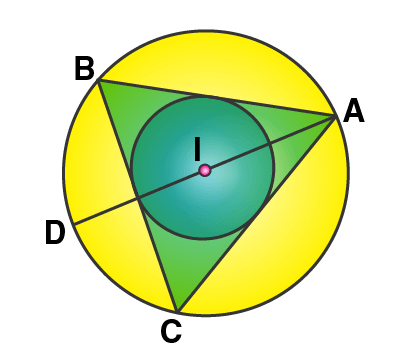Solution: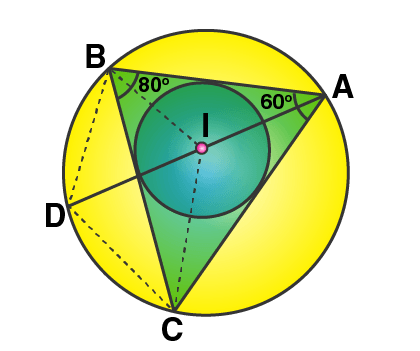Join DB and DC, IB and IC.

Given, if ∠BAC = 66o and ∠ABC = 80o, I is the incentre of the ∆ABC.

(i) As it’s seen that ∠DBC and ∠DAC are in the same segment,

So, ∠DBC = ∠DAC

But, ∠DAC = ½ ∠BAC = ½ x 66o = 33o

Thus, ∠DBC = 33o

(ii) And, as I is the incentre of ∆ABC, IB bisects ∠ABC.

Therefore,

∠IBC = ½ ∠ABC = ½ x 80o = 40o

(iii) In ∆ABC, by angle sum property

∠ACB = 180o – (∠ABC + ∠BAC)

∠ACB = 180o – (80o + 66o)

∠ACB = 180o – 156o

∠ACB = 34o

And since, IC bisects ∠C

Thus, ∠ICB = ½ ∠C = ½ x 34o = 17o

Now, in ∆IBC

∠IBC + ∠ICB + ∠BIC = 180o

40o + 17o + ∠BIC = 180o

57o + ∠BIC = 180o

∠BIC = 180o – 57o

Therefore, ∠BIC = 123o

10. In the given figure, AB = AD = DC = PB and ∠DBC = xo. Determine, in terms of x:

(i) ∠ABD, (ii) ∠APB.

Hence or otherwise, prove that AP is parallel to DB.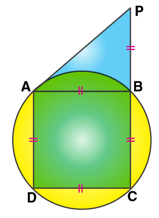Solution: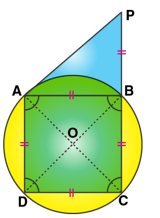Given, AB = AD = DC = PB and ∠DBC = xo

Join AC and BD.

Proof:

∠DAC = ∠DBC = xo [Angles in the same segment]

And, ∠DCA = ∠DAC = xo [As AD = DC]

Also, we have

∠ABD = ∠DAC [Angles in the same segment]

And, in ∆ABP

Ext. ∠ABD = ∠BAP + ∠APB

But, ∠BAP = ∠APB [Since, AB = BP]

2 xo = ∠APB + ∠APB = 2∠APB

2∠APB = 2xo

So, ∠APB = xo

Thus, ∠APB = ∠DBC = xo

But these are corresponding angles,

Therefore, AP || DB.

11. In the given figure; ABC, AEQ and CEP are straight lines. Show that ∠APE and ∠CQE are supplementary.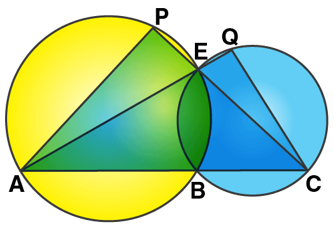Solution: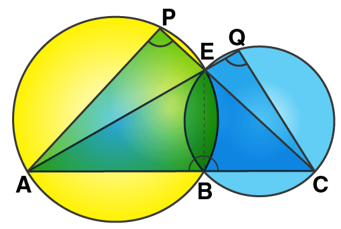Join EB.

∠APE + ∠ABE = 180o ….. (i) [Opposite angles of a cyclic quad. are supplementary]

∠CQE + ∠CBE = 180o ….. (ii) [Opposite angles of a cyclic quad. are supplementary]

Adding (i) and (ii), we have

∠APE + ∠ABE + ∠CQE + ∠CBE = 180o + 180o = 360o

∠APE + ∠ABE + ∠CQE + ∠CBE = 360o

But, ∠ABE + ∠CBE = 180o [Linear pair]

∠APE + ∠CQE + 180o = 360o

∠APE + ∠CQE = 180o

Therefore, ∠APE and ∠CQE are supplementary.

12. In the given, AB is the diameter of the circle with centre O.

If ∠ADC = 32o, find angle BOC.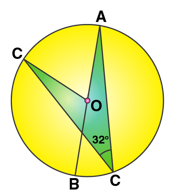Solution:

Arc AC subtends ∠AOC at the centre and ∠ADC at the remaining part of the circle.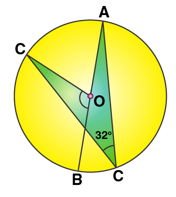Thus, ∠AOC = 2∠ADC

∠AOC = 2 x 32o = 64o

As ∠AOC and ∠BOC are linear pair, we have

∠AOC + ∠BOC = 180o

64o + ∠BOC = 180o

∠BOC = 180o – 64o

Therefore, ∠BOC = 116o